# Predicted time series and residuals#

Here we fit a First Level GLM with the minimize_memory-argument set to False. By doing so, the FirstLevelModel-object stores the residuals, which we can then inspect. Also, the predicted time series can be extracted, which is useful to assess the quality of the model fit.

Note

If you are using Nilearn with a version older than `0.9.0`, then you should either upgrade your version or import maskers from the `input_data` module instead of the `maskers` module.

That is, you should manually replace in the following example all occurrences of:

```from nilearn.maskers import NiftiMasker
```

with:

```from nilearn.input_data import NiftiMasker
```

## Import modules#

```import pandas as pd
from nilearn.datasets import fetch_spm_auditory

subject_data = fetch_spm_auditory()
fmri_img = image.concat_imgs(subject_data.func)

# Make an average
mean_img = image.mean_img(fmri_img)

# Clean and smooth data
fmri_img = image.clean_img(fmri_img, standardize=False)
fmri_img = image.smooth_img(fmri_img, 5.0)

```

## Fit model#

Note that minimize_memory is set to False so that FirstLevelModel stores the residuals. signal_scaling is set to False, so we keep the same scaling as the original data in fmri_img.

```from nilearn.glm.first_level import FirstLevelModel

fmri_glm = FirstLevelModel(
t_r=7,
drift_model="cosine",
signal_scaling=False,
minimize_memory=False,
)

fmri_glm = fmri_glm.fit(fmri_img, events)
```

## Calculate and plot contrast#

```from nilearn import plotting

z_map = fmri_glm.compute_contrast("active - rest")

plotting.plot_stat_map(z_map, bg_img=mean_img, threshold=3.1)
```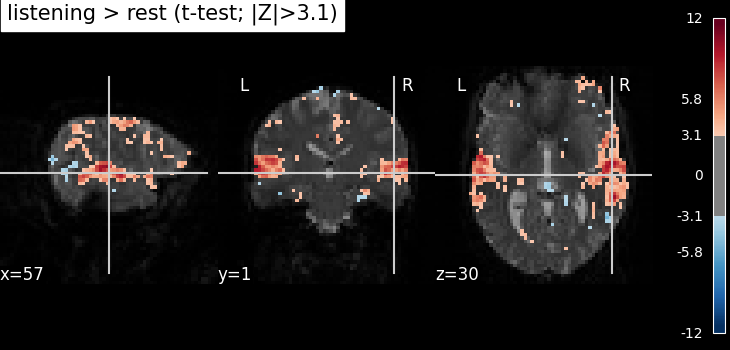```<nilearn.plotting.displays._slicers.OrthoSlicer object at 0x7f7ef08ef2b0>
```

## Extract the largest clusters#

```from nilearn.maskers import NiftiSpheresMasker
from nilearn.reporting import get_clusters_table

table = get_clusters_table(z_map, stat_threshold=3.1, cluster_threshold=20)
table.set_index("Cluster ID", drop=True)

# get the 6 largest clusters' max x, y, and z coordinates
coords = table.loc[range(1, 7), ["X", "Y", "Z"]].values

# extract time series from each coordinate
```

## Plot predicted and actual time series for 6 most significant clusters#

```import matplotlib.pyplot as plt

# colors for each of the clusters
colors = ["blue", "navy", "purple", "magenta", "olive", "teal"]
# plot the time series and corresponding locations
fig1, axs1 = plt.subplots(2, 6)
for i in range(6):
# plotting time series
axs1[0, i].set_title(f"Cluster peak {coords[i]}\n")
axs1[0, i].plot(real_timeseries[:, i], c=colors[i], lw=2)
axs1[0, i].plot(predicted_timeseries[:, i], c="r", ls="--", lw=2)
axs1[0, i].set_xlabel("Time")
# plotting image below the time series
roi_img = plotting.plot_stat_map(
z_map,
cut_coords=[coords[i]],
threshold=3.1,
figure=fig1,
axes=axs1[1, i],
display_mode="z",
colorbar=False,
bg_img=mean_img,
)
fig1.set_size_inches(24, 14)
```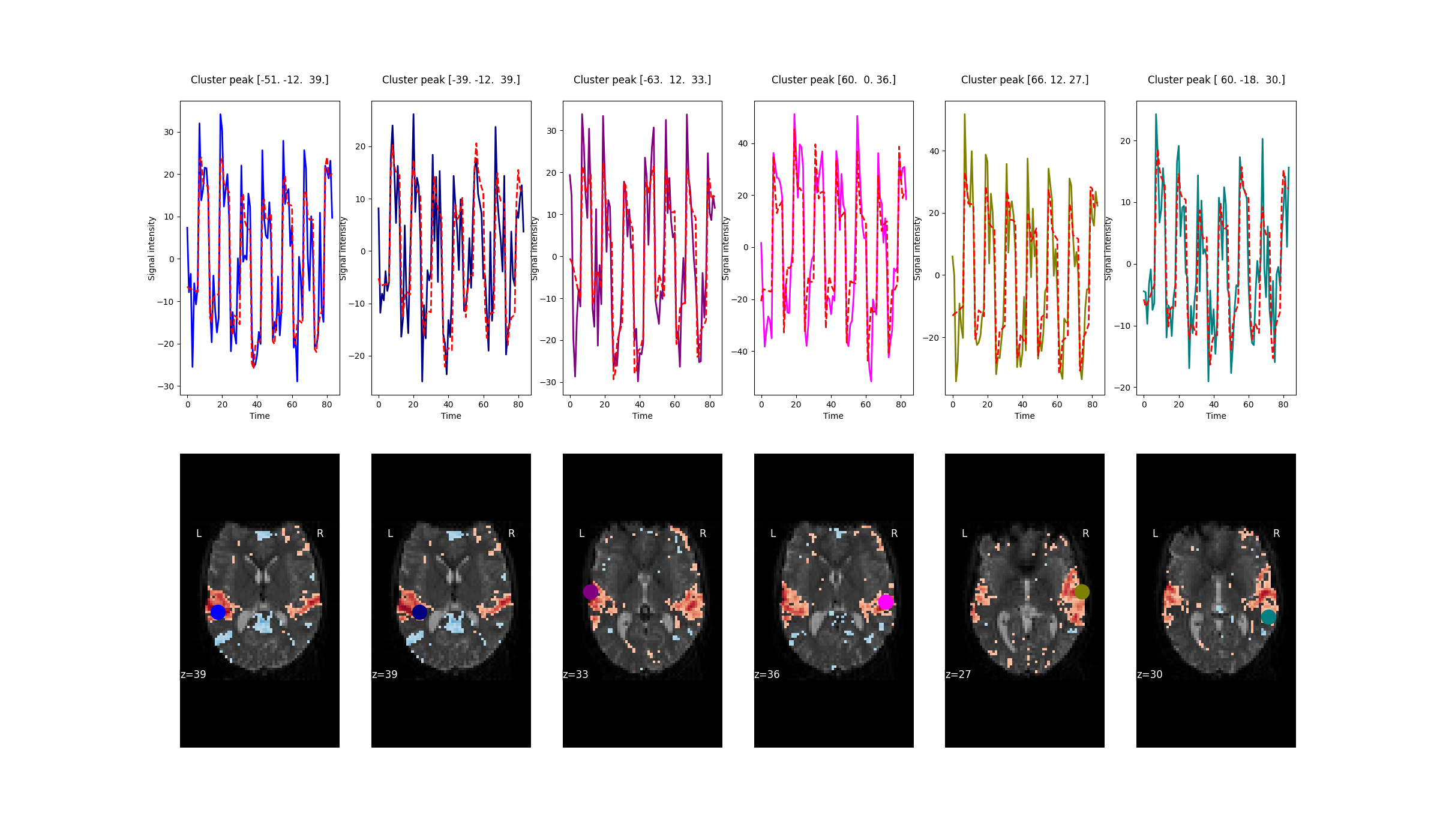## Get residuals#

```resid = masker.fit_transform(fmri_glm.residuals)
```

## Plot distribution of residuals#

Note that residuals are not really distributed normally.

```fig2, axs2 = plt.subplots(2, 3)
axs2 = axs2.flatten()
for i in range(6):
axs2[i].set_title(f"Cluster peak {coords[i]}\n")
axs2[i].hist(resid[:, i], color=colors[i])
print(f"Mean residuals: {resid[:, i].mean()}")

fig2.set_size_inches(12, 7)
fig2.tight_layout()
```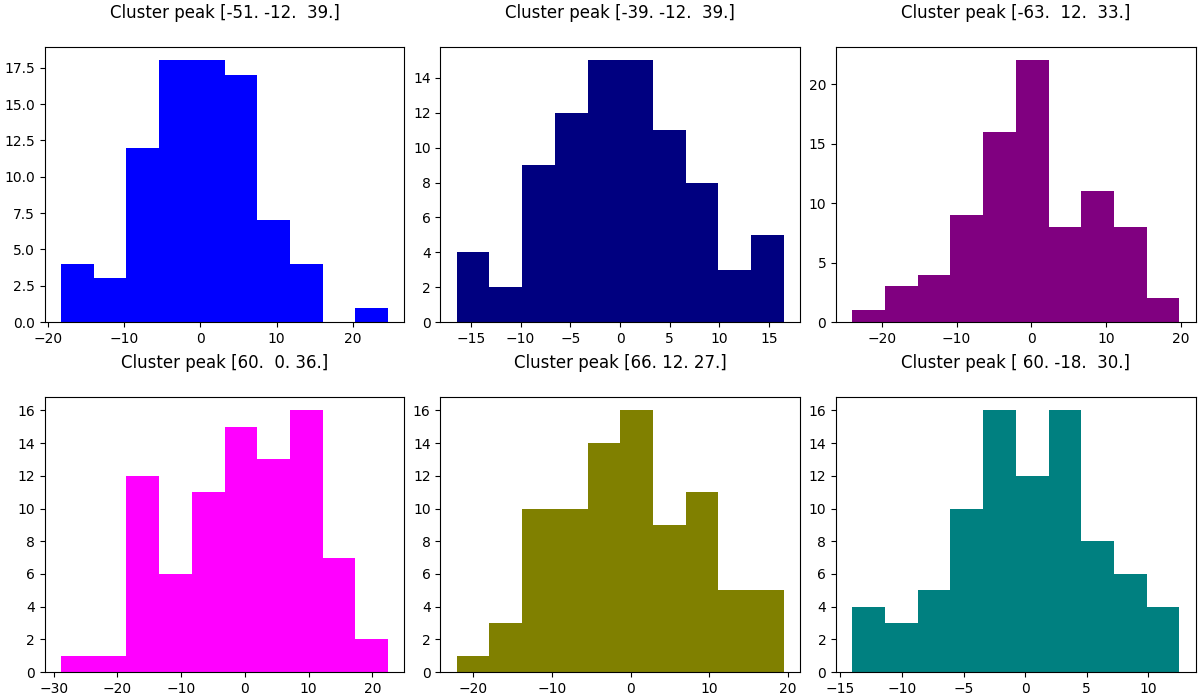```Mean residuals: 0.002374945330088011
Mean residuals: 0.0016934773463647506
Mean residuals: -0.01850990756587893
Mean residuals: -0.07049870657347272
Mean residuals: -0.031008074173812943
Mean residuals: 6.587323279442596e-15
```

## Plot R-squared#

Because we stored the residuals, we can plot the R-squared: the proportion of explained variance of the GLM as a whole. Note that the R-squared is markedly lower deep down the brain, where there is more physiological noise and we are further away from the receive coils. However, R-Squared should be interpreted with a grain of salt. The R-squared value will necessarily increase with the addition of more factors (such as rest, active, drift, motion) into the GLM. Additionally, we are looking at the overall fit of the model, so we are unable to say whether a voxel/region has a large R-squared value because the voxel/region is responsive to the experiment (such as active or rest) or because the voxel/region fits the noise factors (such as drift or motion) that could be present in the GLM. To isolate the influence of the experiment, we can use an F-test as shown in the next section.

```plotting.plot_stat_map(
fmri_glm.r_square,
bg_img=mean_img,
threshold=0.1,
display_mode="z",
cut_coords=7,
)
```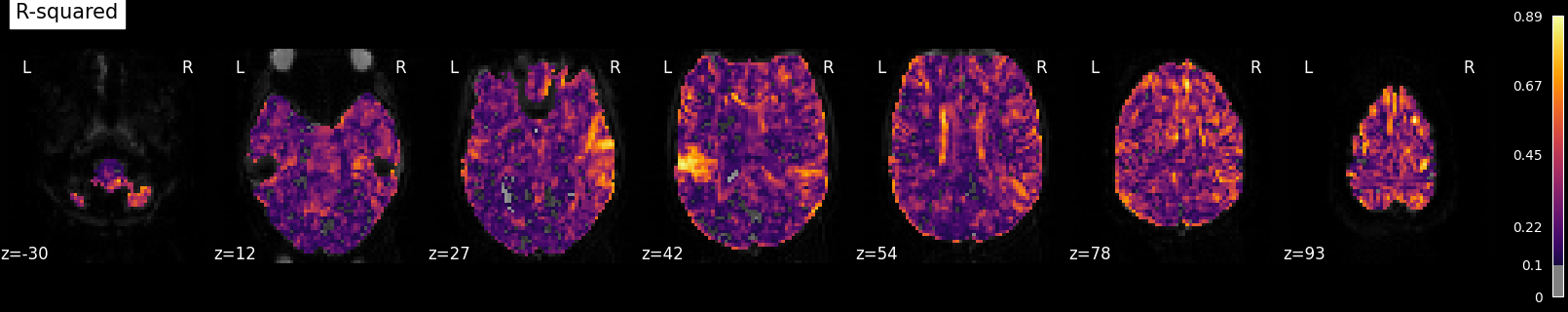```<nilearn.plotting.displays._slicers.ZSlicer object at 0x7f7ef8195b20>
```

## Calculate and Plot F-test#

The F-test tells you how well the GLM fits effects of interest such as the active and rest conditions together. This is different from R-squared, which tells you how well the overall GLM fits the data, including active, rest and all the other columns in the design matrix such as drift and motion.

```import numpy as np

design_matrix = fmri_glm.design_matrices_

# contrast with a one for "active" and zero everywhere else
active = np.array([1 if c == "active" else 0 for c in design_matrix.columns])

# contrast with a one for "rest" and zero everywhere else
rest = np.array([1 if c == "rest" else 0 for c in design_matrix.columns])

effects_of_interest = np.vstack((active, rest))
# f-test for rest and activity
z_map_ftest = fmri_glm.compute_contrast(
effects_of_interest, stat_type="F", output_type="z_score"
)

plotting.plot_stat_map(
z_map_ftest, bg_img=mean_img, threshold=3.1, display_mode="z", cut_coords=7
)
```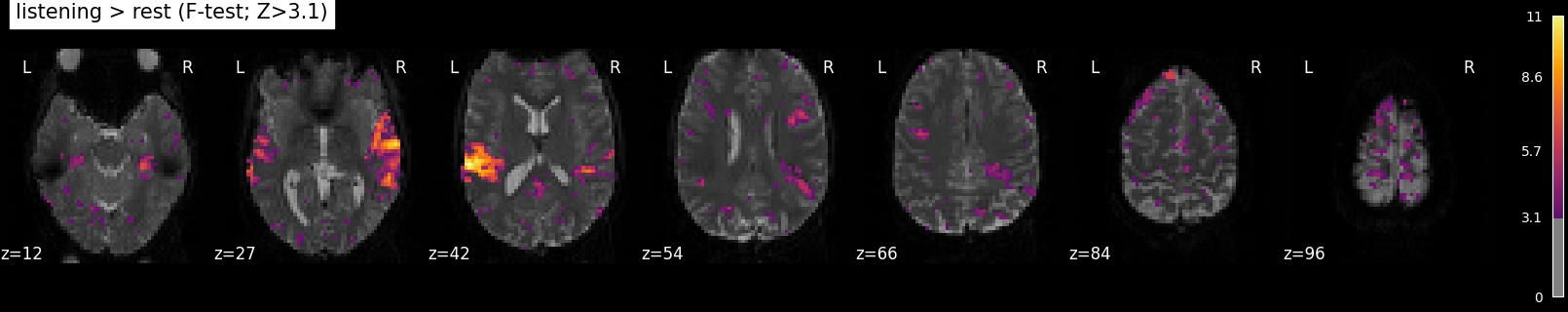```<nilearn.plotting.displays._slicers.ZSlicer object at 0x7f7ee39cf670>
```

Total running time of the script: ( 0 minutes 28.608 seconds)

Estimated memory usage: 645 MB

Gallery generated by Sphinx-Gallery